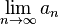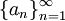# Relationship to Sequences of Absolute Values

Previous: Limit Laws Example

Next: Example Relating Sequences of Absolute Values

The following theorem can be used to evaluate limits of sequences when the sign of the terms in the sequence alternate between positive and negative.

Theorem: Relationship to Sequences of Absolute Values

The limitequals zero if and only ifIn other words, if we are given the sequenceandthenThe converse is also true but we will not use it. For us, this theorem is only useful as a test for convergence.

Previous: Limit Laws Example

Next: Example Relating Sequences of Absolute Values